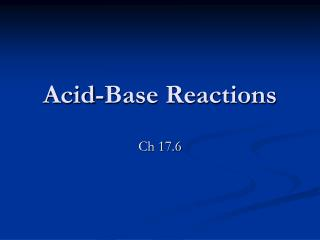DownloadDownload PresentationAcid-Base Reactions

# Acid-Base Reactions

Télécharger la présentation## Acid-Base Reactions

- - - - - - - - - - - - - - - - - - - - - - - - - - - E N D - - - - - - - - - - - - - - - - - - - - - - - - - - -
##### Presentation Transcript

1. Acid-Base Reactions Ch 17.6

2. What kind of reactions are possible? • There are 4 types: • Strong Acid/ Strong Base • Strong Acid/ Weak Base • Weak Acid/ Strong Base • Weak Acid/ Weak Base = no fun

3. Strong Acid and Strong Base • It is easy since it is 100% Ionized • The Net Ionic is just the hydronium ion with the hydroxide ion to make water • Very Large K Value = All product (for all practical purposes.

4. Strong acid is completely ionized in solution to form hydronium ion The hydronium ion reacts with the OH ions from the weak base. Ex: HCl and Ammonia Large K value = goes essentially to completion NH4+ is the conjugate acid of a weak base so it creates an Acid in water. Strong Acid w/ a Weak Base

5. Important Ideas here! • Mixing equal molar quantities of a strong acid and a weak base produces an acidic solution • The overall reaction is the reverse that defines Ka for the conjugate acid of the weak base, so the overall K is 1/ Ka. NH4+ ‘s Ka = 5.6 X 10-10 so Knet = 1/(5.6 X 10-10 ) = 1.8 X 109

6. Weak Acid with a Strong Base • Take every thing we said and reverse it • Ti esrever dna dias ew gniht yreve ekat • Equal molar quantities makes a basic solution • The conjugate base of the weak acid’s Kb is related to the Knet = 1/Kb

7. Weak Acid with a Weak Base • If they are equal volumes, look at the relative Ka and Kb’s to determine • Acetic acid mixed with ammonia

8. Solving for the pH of an Acid base solution • If you add 50mL of 0.10M NaOH with exactly 50mL of 0.1M formic acid HCO2H. What is the pH of the resulting solution? Kb is 5.6 X 10-11 • Step 1: Do the Stoich • Calculate the quantity of base added, quantity of acid consumed, and the quantity of conj base formed • Step 2: Calc concentrations Calculate the conc of conj base (VOLUMES ARE ADDITIVE) • Find pH using Kb of cong base